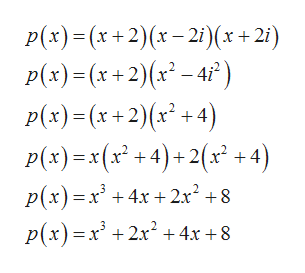O POLYNOMIAL AND RATIONAL FUNCTIONSFinding a polynomial of a given degree with given zeros: Comple...Find a polynomial f(x) of degree 3 with real coefficients and the following zeros.-2, 2if(x)

Question

see attachmenthelp_outlineImage TranscriptioncloseO POLYNOMIAL AND RATIONAL FUNCTIONS Finding a polynomial of a given degree with given zeros: Comple... Find a polynomial f(x) of degree 3 with real coefficients and the following zeros. -2, 2i f(x) fullscreen
Step 1

We know that if a+ib is zero of a polynomial, then a-ib is also its polynomial. So, zeros of the required polynomial are -2,2i,-2i

Step 2

Thus the polynomial has facto...help_outlineImage TranscriptioncloseP(x)(x+2)x-21)(x+2i p(x) (x+2)(x-4) p(x)(x2)x +4) P(x) x(x +4)+2(x+4) p(x) x 4x+2x 8 p(x)x2x24x +8 fullscreen

Want to see the full answer?

See Solution

Want to see this answer and more?

Our solutions are written by experts, many with advanced degrees, and available 24/7

See Solution
Tagged in

Other